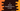# C program to check if a Hexadecimal number is even or odd## C program to check if a Hexadecimal number is even or odd:

A number is called even if it is devisible by 2 and odd if it is not. This is same for a hexadecimal number as well. The trick is to check if a hexadecimal number can be divided by 2 or not.

In this post, we will write one C program that will check if a hexadecimal number is even or odd.

### How to check if a Hexadecimal number is even/odd:

For any number, we can check its last digit and find out if it is even or odd. We can check if the last digit of a number is divisible by 2 or not. If it is divisible, it is an even number, else odd.

Hexadecimal numbers are 16 base numbers and one number is defined using 0 to 9 and A to F. We can say that a hexadecimal number is even if it ends with 0, 2, 4, 6, 8, A, C or E. A stands for 10, C stands for 12 and E for 14.

We can check the last value of a hexadecimal number and we can say it is odd or even.

### C program for hexadecimal even/odd check:

Let’s write the program in C :

``````#include <stdio.h>
#include <string.h>

int isEven(char *hexa, int length)
{
switch (hexa[length - 1])
{
case '0':
return 1;
break;
case '2':
return 1;
break;
case '4':
return 1;
break;
case '6':
return 1;
break;
case '8':
return 1;
break;
case 'A':
return 1;
break;
case 'C':
return 1;
break;
case 'E':
return 1;
break;
default:
return -1;
break;
}
}
int main()
{
char *thousand = "64E";
if (isEven(thousand, strlen(thousand)) == -1)
{
printf("Odd Number");
}
else
{
printf("Even Number");
}
}``````

### Explanation:

In this program:

• isEven method is used to check if a hexadecimal number is even or odd. It uses one switch statement to determine the last character.
• length is the length of the string that we are passing to isEven method.
• It returns -1 for odd numbers and 1 for even.

For the above program, it will print the below output:

``Even Number``

You can also try to run this program with different hexadecimal values and check the result.